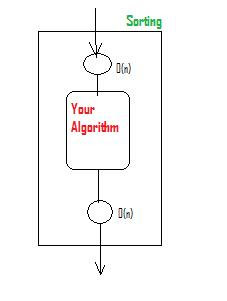## Friday, May 09, 2008

### P versus NP

Its an unsolved problem in computer Science. Infact, if you could find a solution to it, you will get a million dollars. Well, I am not kidding (http://www.claymath.org/millennium/).
Now aren't you curious ?

Here is the official problem description: http://www.claymath.org/millennium/P_vs_NP/Official_Problem_Description.pdf

I will try to explain it to you in more simpler way. It is extremely titillating problem because it is very simple to understand it and first time when you hear it, it seems that you can crack it witihin a day or two.

I will assume few things:You know what an algorithm is and what is worst, average and best case complexities (asymtotic notations) are. You may want to refer to Chapter 1,2 and 3 in Introduction to Algorithm by Cormen

Section 1: Lower bound
Say you are given a problem and you find an algorithm that solves that problem in O(n^2) time. What is the lower bound of your algorithm (or should I say the problem -- Hmmm, I will use algorithm and the problem interchangeably) ?
If your answer is O(n^2), you may want to read this section further, else you can skip this and go to the next section.

Lower bound is not about a particular algorithm, but about all possible algorithms for solving that problem.
I know 2 ways to find lower bound of the algorithms:

1. Decision tree based approach:
Eg: Lower bound of Comparison based Sorting (See this: http://www.ics.uci.edu/~eppstein/161/960116.html)

In short, comparison sort can be mapped to a decision tree (binary tree with yes and no). Longest path in binary tree with k leaves = log k.Since we know a number can be arranged in n! ways => n! leaves of decision trees.There, number of comparisions = log(n!) = n log(n) - O(n) .... using Stirling's formula.

2. Reduction:
Reduce an algorithm whose lower bound you already know to your algorithm (Thats right ... not the other way round).
Eg. Say you somehow prove that Sorting <= (O(n)) Ur algorithm, ie. Sorting is reducible to your algorithm in polynomial timeThen you can state that lower bound of your algorithm is nlogn.This in essense means that your algorithm is atleast as difficult as sorting. So, how to prove this ? Prove that by some crazy way, "Sorting can be done by your algorithm"Section 2: Definitions
Decision problems: Problem with output either "YES" or "NO", depending on values of some input parameters

P: Class of decision problems that are "solvable" in polynomial time.

NP: Class of the decision problem that can be "verified" in polynomial time.
Eg: Sorting is NP since given a list, we can verify that it is sorted or not in polynomial time.Also, Sorting is P since it is solvable in polynomial time (O(nlogn)).
Infact, Every P problem is NP (ie to verify a P problem, we can simply solve it in polynomial time and compare the input with the output). i.e P belongsto NPWhether there exists any NP problem that is not P is yet unproven.
Trivial Solution - Every NP problem can be solved in 2^n, ie simply enumerating all cases.

NP-hard: For all problems L in NP, if L is reducible to L1 in polynomial time, then L1 is NP-hard.
NP-Complete: A problem is NP-Complete iff it is both NP and NP-hard.

See this Venn diagram for big picture.

co-NP: If complement of your problem is in NP, then your problem is in co-NP.
Eg: NP - Given a graph, is there a path of length less than k from some source node s to destination node t.co-NP - Given a graph, are all path of length greater than k
Again, whether NP = co-NP is unknown ?

Oracle: Consider Oracle to be GOD who knows everything and whom you can trust (So, computer scientist believe in GOD ... may be not in way as religion, but maybe yes to some extent)

We "pray" to GOD to give guidance. Similarly, we ask Oracle to help us with the problem.

GOD give us omens. Oracle gives us a certificate (an input sequence that will probably reveal the "TRUTH"). So you try to verify the certificate by your polynomial time verification algorithm (assuming it is NP).

Now here is a trick:

If the answer to that certificate is "YES" ... cool ... you have solved the problem !!!

If the answer is "NO" .... you ask for a new certificate from the Oracle.

(You could argue that since we trust Oracle, we should output "NO". But, we don't do that because Oracle gives a random certificate for undecidable problems. This makes it indeterministic, hence NP => nondeterministic polynomial).

Section 3: The real stuff
The biggest breakthrough in this area: Stephen Cook proved that Boolean Satisfiability problem (SAT) is NP-Complete.

So, if you could prove one of this things, you probably don't have to work any more for your thesis or field's medal:
1. SAT has polynomial time algorithm
2. Give non-polynomial time lower bound argument for SAT

If you prove 1 => you have proven P = NP
If you prove 2 => you have proven P != NP

OK. Let me makes things a little easy for you. Instead of SAT, you can do above things on any one of these problems

And yippee .... you will be more popular than Donald Knuth in Computer Science field.

I would encourage you to read "Computers and Intractability: A Guide to the Theory of NP-Completeness" or atleast a chapter in "Introduction to Algorithms - by Cormen, Rivest" to see how reduction actually works. Some of them are really cool and mind boggling techniques.